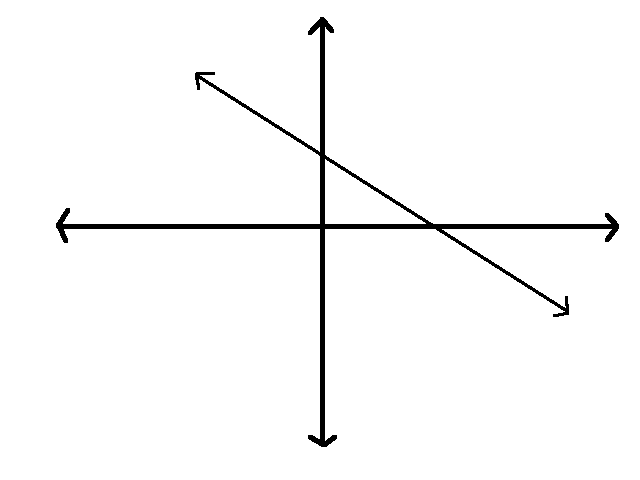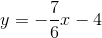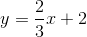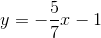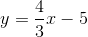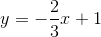# PSAT Math : How to graph a line

## Example Questions

### Example Question #1 : Graphing

A line graphed on the coordinate plane below.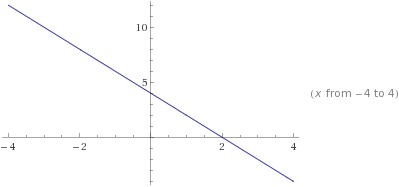Give the equation of the line in slope intercept form.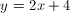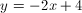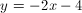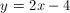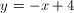Explanation:

The slope of the line is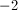and the y-intercept is.

The equation of the line is### Example Question #665 : Sat Mathematics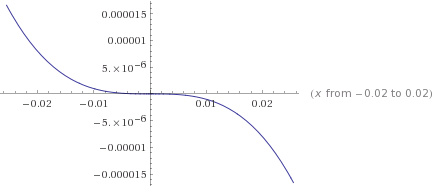Give the equation of the curve.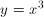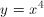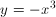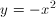Explanation: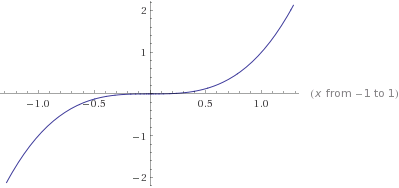This is the parent graph of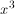. Since the graph in question is negative, then we flip the quadrants in which it will approach infinity. So the graph ofwill start in quadrant 2 and end in 4.

### Example Question #1 : How To Graph A Line

The equation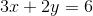represents a line.  This line does NOT pass through which of the four quadrants?

I

Cannot be determined

III

II

IV

III

Explanation:

Plug inforto find a point on the line: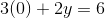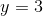Thus,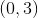is a point on the line.

Plug inforto find a second point on the line: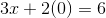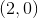is another point on the line.

Now we know that the line passes through the pointsand.

A quick sketch of the two points reveals that the line passes through all but the third quadrant.

### Example Question #2 : Graphing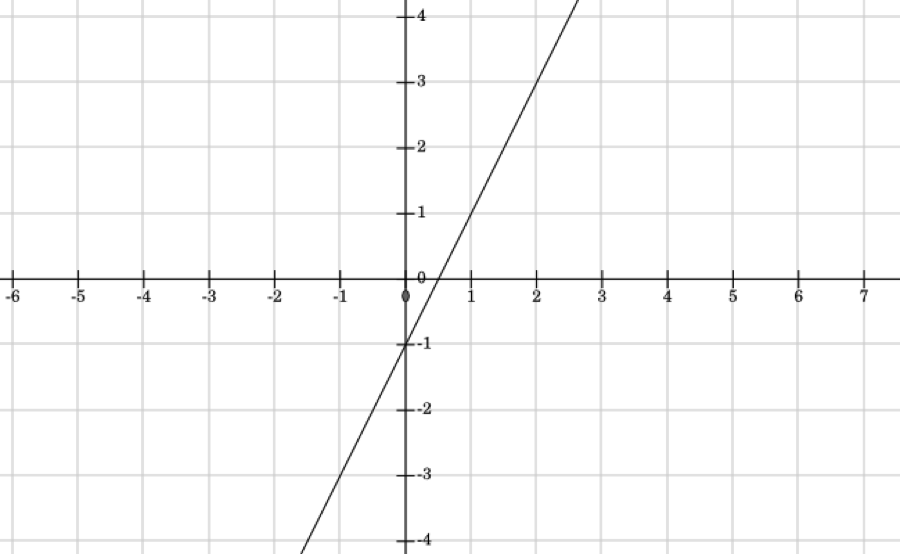What is the equation of the line in the graph above?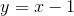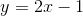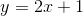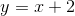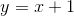Explanation:

In order to find the equation of a line in slope-intercept form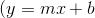, whereis the slope andis the y-intercept), one must know or otherwise figure out the slope of the line (its rate of change) and the point at which it intersects the y-axis. By looking at the graph, you can see that the line crosses the y-axis at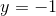.  Therefore,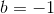.

Slope is the rate of change of a line, which can be calculated by figuring out the change in y divided by the change in x, using the formula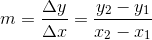.

When looking at a graph, you can pick two points on a graph and substitute their x-  and y-values into that equation.  On this graph, it's easier to choose points like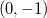and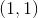.  Plug them into the equation, and you get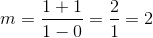Plugging in those values forandin the equation, and you get### Example Question #3 : Graphing

What are the x- and y- intercepts of the equation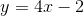?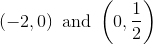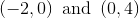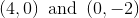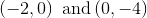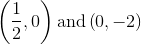Explanation:

Finding the y-intercept: The y-intercept is the point at which the line crosses tye y-axis, meaning that x = 0 and the format of the ordered pair is (0,y) with y being the y-intercept.  The equationis in slope-intercept () form, meaning that the y-intercept, b, is actually given in the equation.  b = -2, which means that our y-intercept is -2.  The ordered pair for expressing this is (0,-2)

Finding the x-intercept: To find the x-intercept of the equation, we must find the point where the line of the equation crosses the x-axis.  In other words, we must find the point on the line where y is equal to 0, as it is when crossing the x-axis.  Therefore, substitute 0 into the equation and solve for x: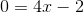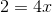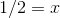The x-interecept is therefore (1/2,0).

### Example Question #4 : Graphing

Which of the following could be the equation of the line shown in this graph?